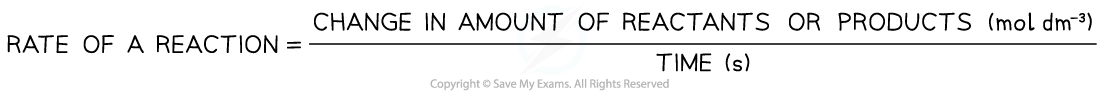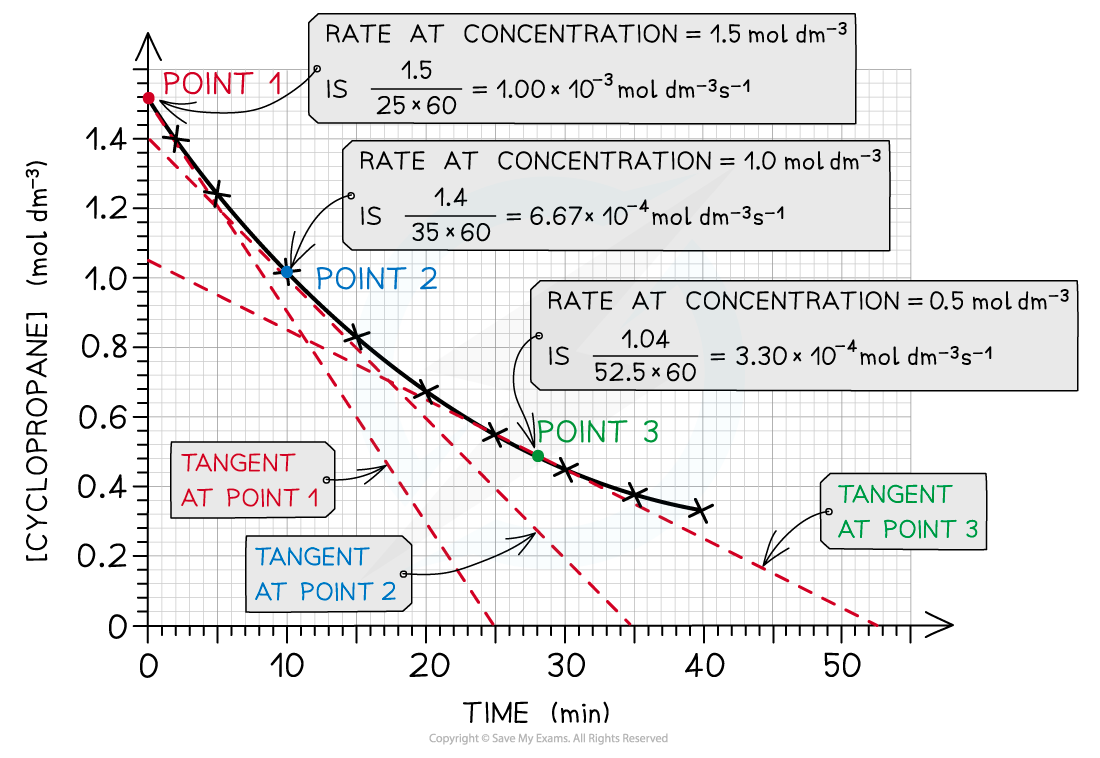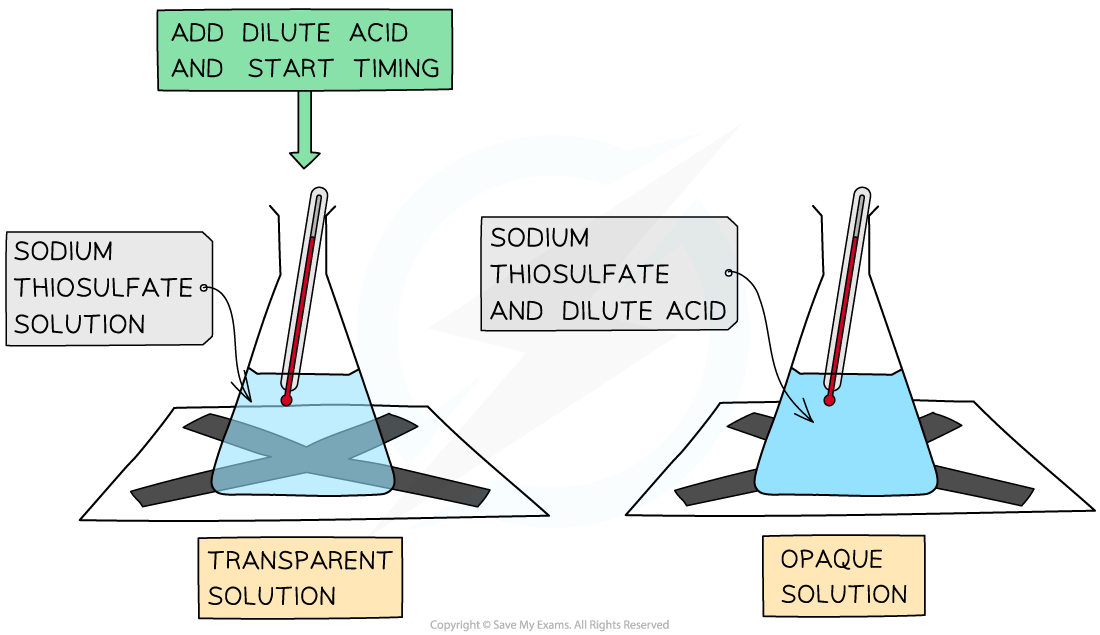# AQA A Level Chemistry复习笔记4.1.4 Factors Affecting the Rate of a Reaction

### Rate of Reaction

#### Factors which affect the rate of a chemical reaction

• During a reaction, the reactants are used up and changed into the products
• The rate of a chemical reaction can be calculated using the following equation:• As a reaction proceeds, the concentration of the reactants is decreasing and the concentration of the products is increasing
• Because of this, the rate of the reaction is not the same throughout the reaction but changes
• The rate of reaction during the reaction can be calculated from a concentration-time graph
• A tangent will have to be drawn at a certain point on the graph, to calculate the rate at that specific time
• The following graph is an example of cyclopropane being converted to propene
• The concentration of cyclopropene, the reactant, is decreasing over time as the reaction progressesThe rate of reaction at 3 different concentrations of cyclopropane is calculated by drawing tangents at those points in the graph

#### The Disappearing Cross Experiment

• A simple experiment which can be done to determine how rate of reaction is affected by concentration is the disappearing cross experiment
• It is set up as follows:The Disappearing Cross Experiment

• This experiment can be done for a number of different reactions, but taking the following reaction as an example:

Na2S2O3 (aq) + 2HCl (aq)  → 2NaCl (aq) + H2O (l) + SO2 (g) + S (s)

• In this reaction, sodium thiosulphate reacts with hydrochloric acid
• The key product which allows this experiment to work, is the sulfur which is a solid, and causes the solution to become opaque
• There are actually two factors which can be investigated using the disappearing cross reaction:
• Changing the temperature
• Changing the concentration of the HCl

• You can plot graphs of concentration against time if the concentration of HCl was changed, or plot 1/t against temperature if the temperature was changed
• You plot 1/t because the rate is proportional to 1/time and the graph you want is rate versus temperature

#### Exam Tip

The disappearing cross experiment can be used for any reaction where a solid is produced as one of the products, as this will cause the solution to become cloudy. It does not have to be done in a conical flask, it could be done in a test tube with the cross placed underneath the test tube rack. Watch out: this is a very simple experiment, but exam questions could try and make this seem more complicated than it is!